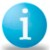# Error propagation

Six Sigma – iSixSigma Forums Old Forums General Error propagation

Viewing 1 post (of 1 total)
• Author
Posts
• #47560Pipkin
Participant

Scenerio:  An N parameter model has been fit to M experimental data points. The residual sum of squared deviations between the data and model (SSQ) yields a regression error Sr=(SSQ/N-M))^1/2. Furthermore, we may compute the prediction uncertainty associated with parameter uncertainty by error propagation using the estimated parameter error covariance matrix. For simplicity, assume the latter is constant for all data points at Sp=0.5 while Sr=0.25.
Question: Should the total prediction uncertainty be computed St = Sr + Sp =0.75 or as (Sr^2 + Sp^2))^1/2 = 0.56 and what is the assumption implicit in either formula? The usual logic for adding variances does not apply (i.e., we are not dealing with the addition of independent parameters but rather with independent sources of error). If we add variances, the result implies that total error is attenuated by considering both error sources, which makes no physical sense to me. Can anyone provide a theoretical rationale for the correct handling of this problem?

0
Viewing 1 post (of 1 total)

The forum ‘General’ is closed to new topics and replies.